CBSE Class 10 Sample Paper for 2022 Boards - Maths Basic [Term 2]

Class 10
Solutions of Sample Papers for Class 10 Boards

## Draw a pair of tangents to a circle of radius 6cm which are inclined to each other at an angle of 600 . Also find the length of the tangent.

This question is similar to Ex 11.2, 4 Chapter 11 Class 10 - Constructions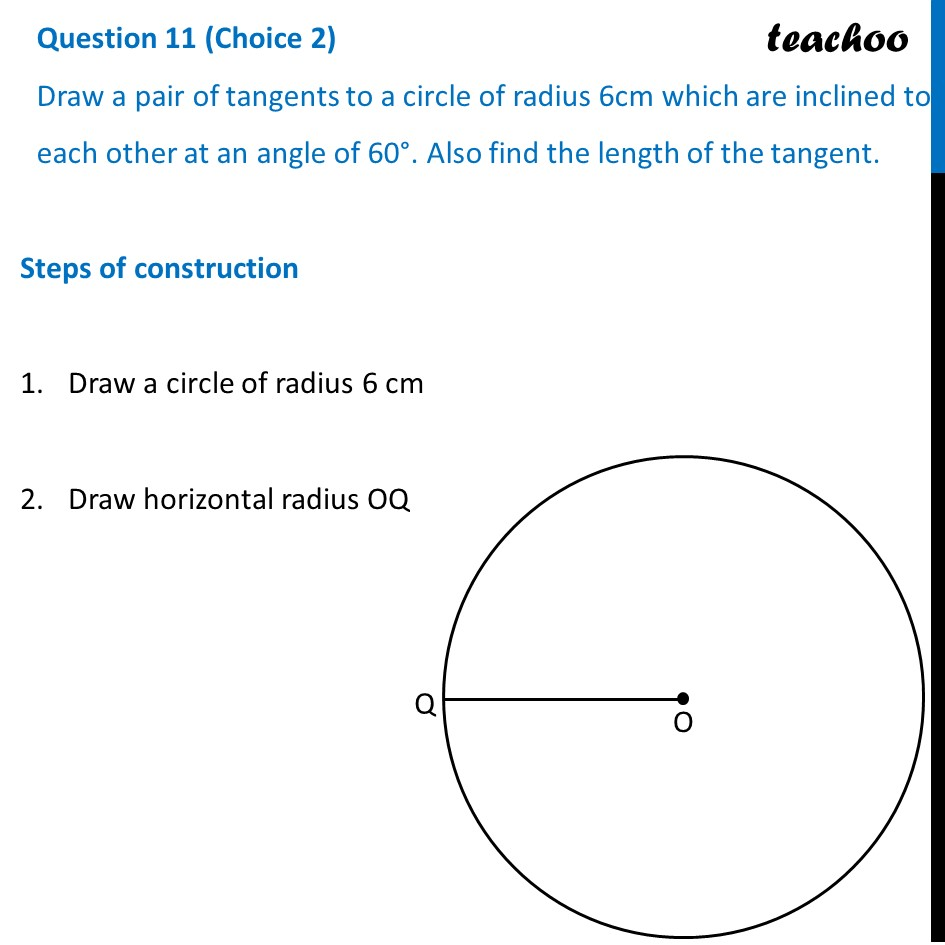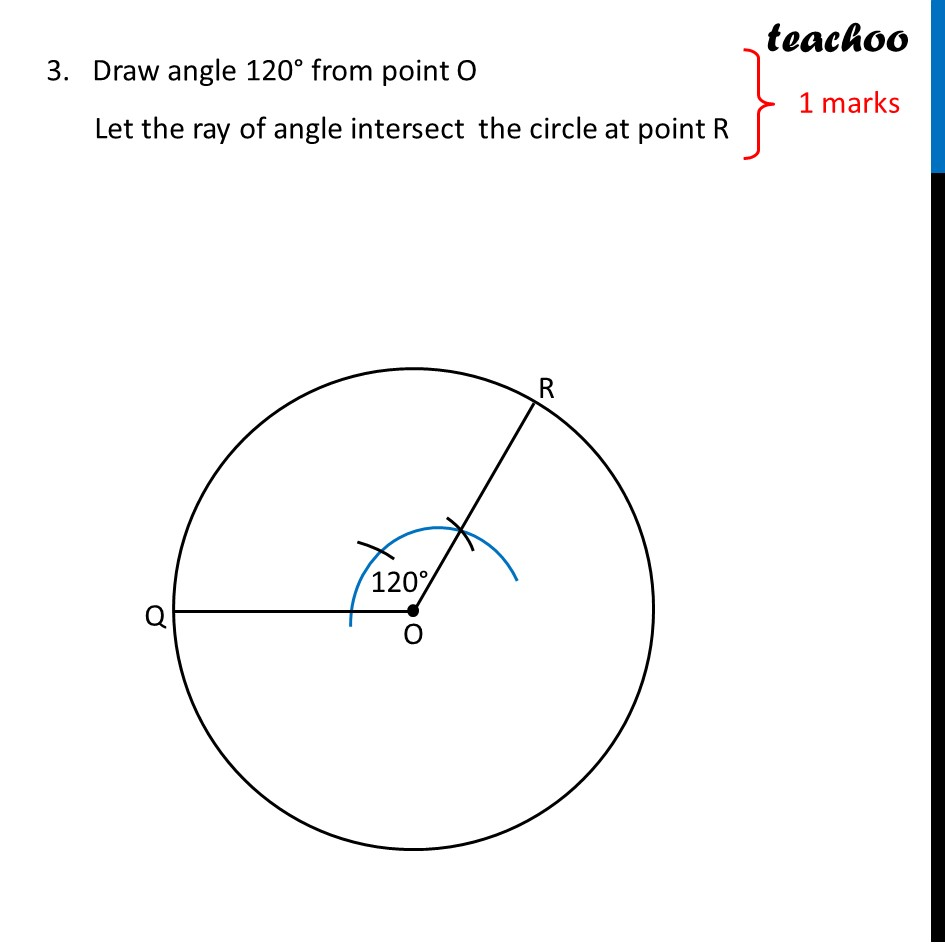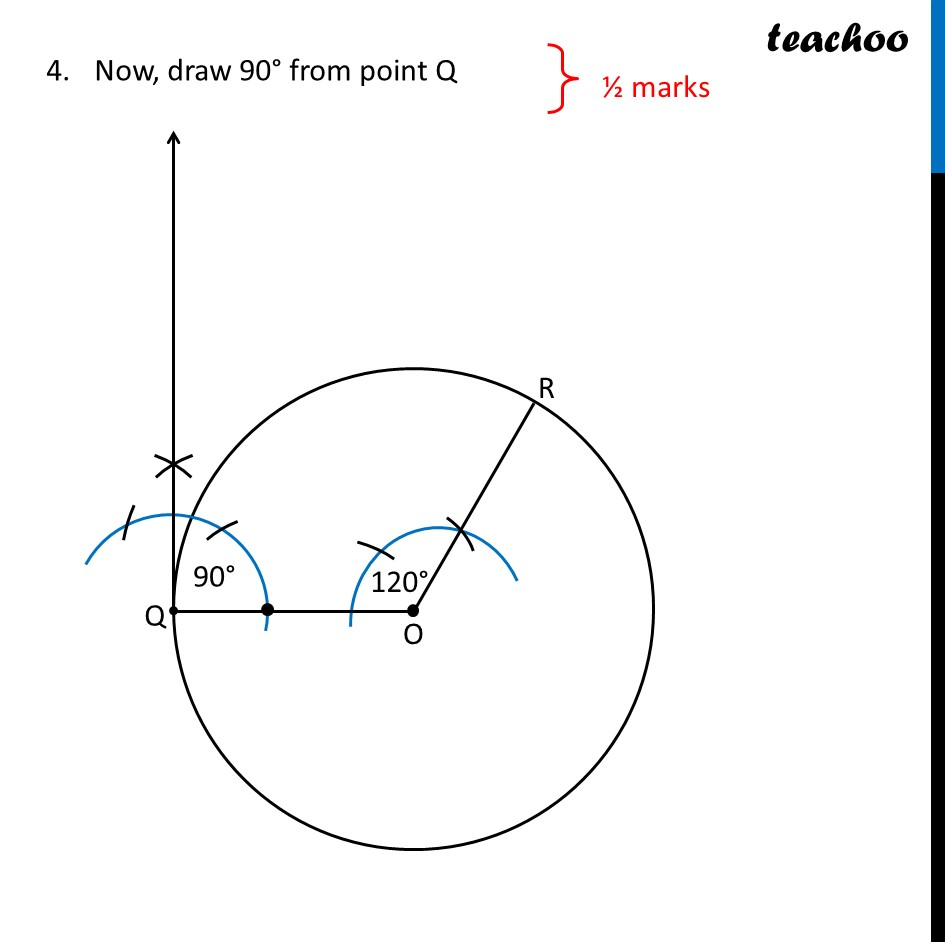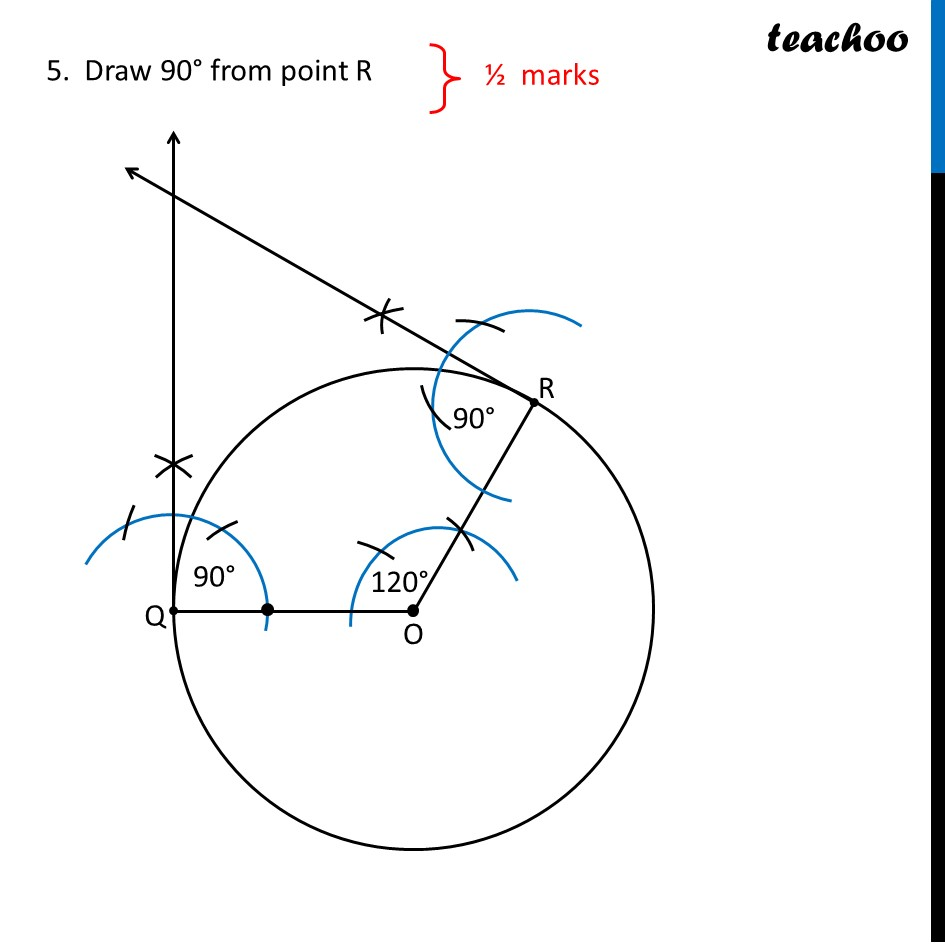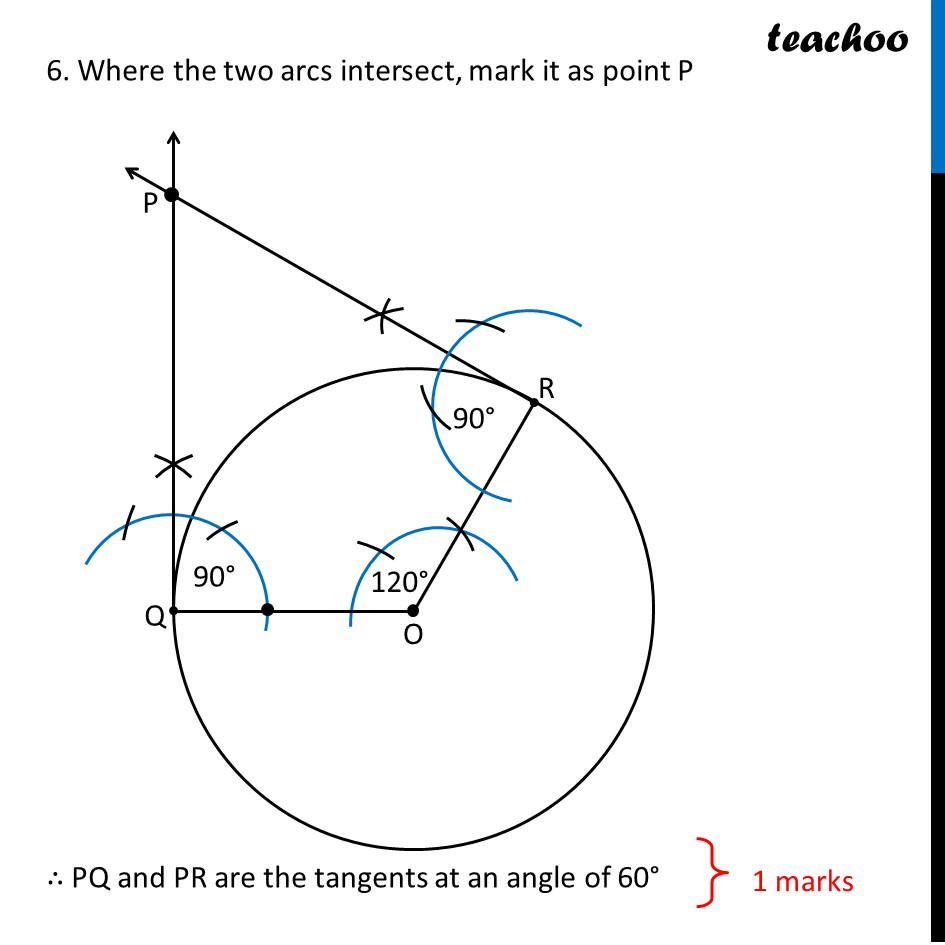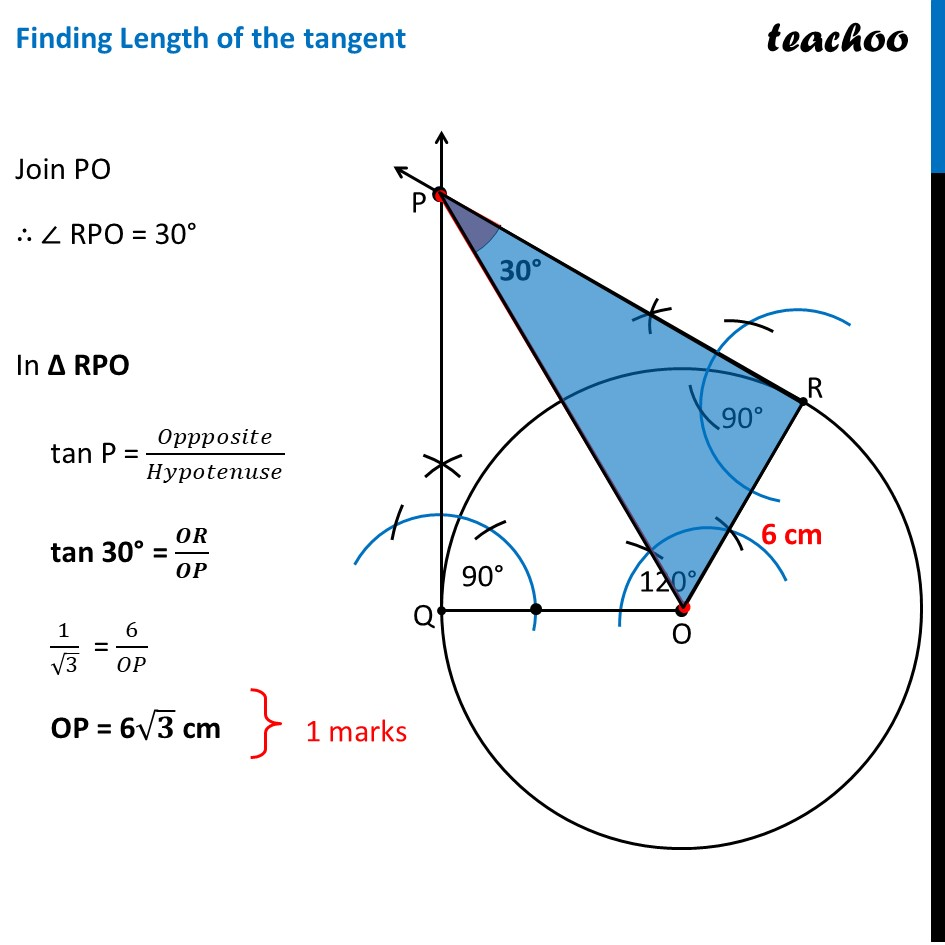Learn in your speed, with individual attention - Teachoo Maths 1-on-1 Class

### Transcript

Question 11 (Choice 2) Draw a pair of tangents to a circle of radius 6cm which are inclined to each other at an angle of 60°. Also find the length of the tangent. Steps of construction Draw a circle of radius 6 cm Draw horizontal radius OQ 3. Draw angle 120° from point O Let the ray of angle intersect the circle at point R Now, draw 90° from point Q 5. Draw 90° from point R 6. Where the two arcs intersect, mark it as point P ∴ PQ and PR are the tangents at an angle of 60° Finding Length of the tangent Join PO ∴ ∠ RPO = 30° In Δ RPO tan P = 𝑂𝑝𝑝𝑝𝑜𝑠𝑖𝑡𝑒/𝐻𝑦𝑝𝑜𝑡𝑒𝑛𝑢𝑠𝑒 tan 30° = 𝑶𝑹/𝑶𝑷 1/√3 = 6/𝑂𝑃 OP = 6√𝟑 cm